## 08 May Java Decision Making Statements

Decision making in Java comprises one or more conditions to be evaluated by the program. Java decision making statements, include the following,

• if statement
• if-else statement
• If-else if-else statement
• Nested Ifs
• Switch Statement
• Nested Switch

Let us discuss them one by one,

## if

Under if, the statement executes if the condition is true. It is the most commonly used decision-making statement in C, C++ and Java.

### Example

Let us see an example, wherein we will learn about the usage of if statement in Java,

### Output

The following is the output,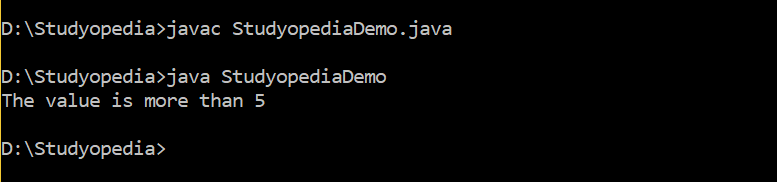## if-else

Under the if-else statement, the first statement executes if the condition is true, else statement two executes.

### Example

Let us see an example, wherein we will learn about the usage of if-else statement in Java,

Output

The following is the output,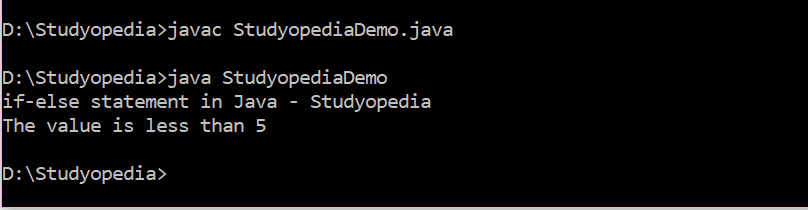## if-else if-else

The if-else if-else executes and whichever condition is true, the associated statement executes. If none of them is true, then else executes.

### Example

Let us see an example, wherein we will learn about the usage of if-elseif-else statement in Java,

Output

The following is the output,## Nested ifs

Under nested ifs, as the name suggests, ifs are nested. If the condition under the topmost if statement is true, then the nested if execute.

### Example

Let us see an example, wherein we will learn about the usage of nested ifs in Java,

### Output

The following is the output,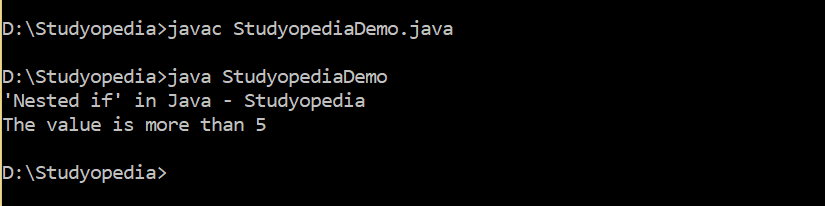## Switch

Under switch statement, the comparison is between the expression and the list of values (case). A match would allow the case statement specific to that value to execute. A break statement is useful inside a switch to terminate a statement sequence.

If none of the value matches with the expression, the default statement executes. However, remember that the default statement is optional.

### Syntax

Here, the expression is a Boolean expression,

### Example

Let us see an example, wherein we learn about the usage of switch statement in Java,

### Output

The following is the output,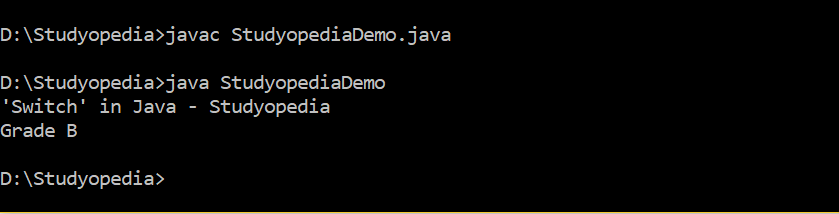## Nested switch

A nested switch occurs when a switch statement is inside another switch. It allows you to add multiple options to a case value.

For example, do you like, “Desktop”, “Laptop”, or “iPad”. If you like laptop, further options can be added i.e. Business Laptops, Gaming Laptops, etc. The flow would be like,Let us see the syntax,

### Example

Let us see an example, wherein we learn about the usage of nested switch,

### Output

The following is the output,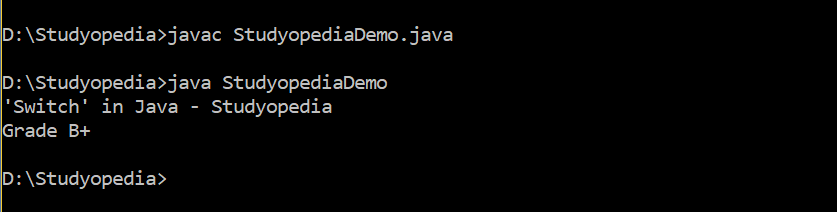In this lesson, we learned how to work with Java Decision Making Statement, such ifs, if-else, switch, etc. In the next lesson, we will learn about loops in Java.

##### Tags:[email protected]

We work to create programming tutorials for all.